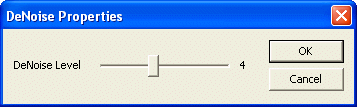﻿ DeNoise Filter

# DeNoise Filter

The DeNoise filter reduces noise in the frames by averaging a number of frames.

## Using the DeNoise Filter

The DeNoise filter is loaded by an application using the following code:

```[C#]FrameFilter DeNoiseFilter;
DeNoiseFilter = ICImagingControl1.FrameFilterCreate("DeNoise", "");
if( DeNoiseFilter == null )
MessageBox.Show("Failed to create DeNoiseFilter");
else

```

## Parameters

The only parameter of the DeNoise filter is the DeNoise Level:

DeNoise Level
The parameter DeNoise Level determines the number of frames to be averaged.
Note: The actual number of frames that are averaged is 2^n, so when setting DeNoise Level to 2, 4 frames are averaged.
This parameter can only be set while the live video stream is stopped.

## Property Dialog

The number of frames to be averaged can be adjusted in the filter's property dialog:## Programmatically Access the Parameter

The following source code can be used, if the parameter should be set by an application:

```[C#]// Retrieve the current denoise level.
int level = DeNoiseFilter.GetIntParameter("DeNoise Level");
// Set a new denoise level.
level = 3;
if( !ICImagingControl1.LiveVideoRunning )
{
// A new denoise level can only be set, while the live video is stopped.
DeNoiseFilter.SetIntParameter("DeNoise Level2", level);
}

```

<< IC Imaging Control Standard Filters